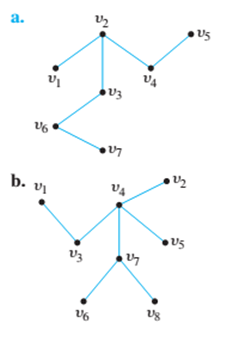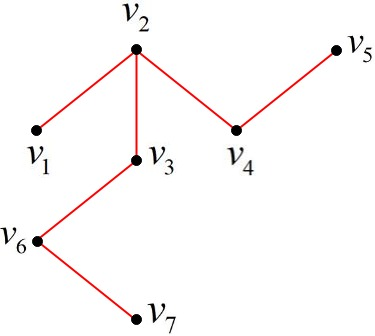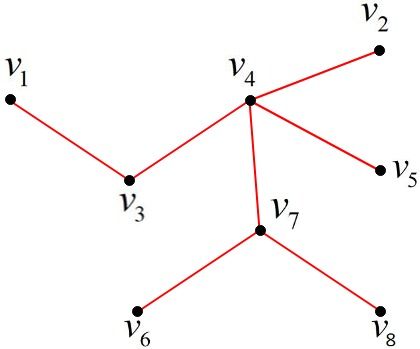Chapter 10.4, Problem 7ES### Discrete Mathematics With Applicat...

5th Edition
EPP + 1 other
ISBN: 9781337694193

#### Solutions

Chapter
Section### Discrete Mathematics With Applicat...

5th Edition
EPP + 1 other
ISBN: 9781337694193
Textbook Problem
8 views

# Find all leaves (or terminal vertices) and all internal (or branch) vertices for the following tree.To determine

(a)

To find:

All terminal vertices and all internal vertices for the given tree.Explanation

Given information:

The diagram has 7 vertices v1,v2,v3,v4,v5,v6 and v7.

Concept used:

If a tree has one or two vertices, then each vertex is called a terminal vertex.

If a tree has at least 3 vertices, then the vertex of degree 1 in the tree is called the terminal vertex.

A vertex or degree greater than 1 in the tree is called an internal vertex.

The degree of a vertex is equal to the number of edges that are incident on the vertex.

The edge which is part of a loop is counted twice.

The sum of the degrees of all the vertices of the tree graph is called the total degree of the graph.

The degree of a vertex is denoted as deg (vertex)

Calculation:

The degree of the vertices v1,v2,v3,v4,v5,v6 and v7 are shown below.

The degree or vertex v1 in 1, since only one edge is incident on vertex v1.

The degree or vertex is v2 is 3, since 3 edges are incident on vertex v2.

The degree or vertex v3 is 2, since 2 edges are incident on vertex v3

To determine

(b)

To calculate:

All terminal vertices and all internal vertices for the specified tree.### Still sussing out bartleby?

Check out a sample textbook solution.

See a sample solution

#### The Solution to Your Study Problems

Bartleby provides explanations to thousands of textbook problems written by our experts, many with advanced degrees!

Get Started